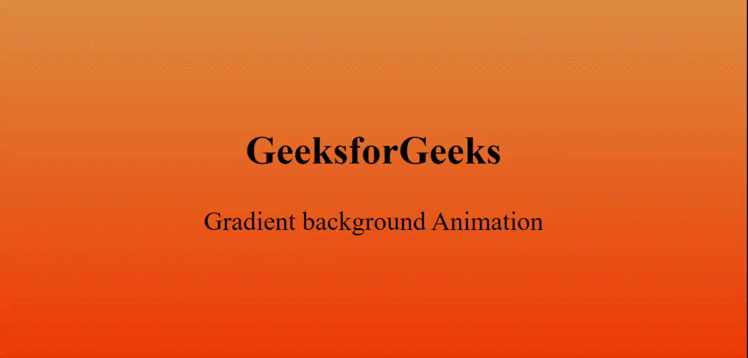# How to Create Gradient Background Animation using HTML and CSS ?

Gradient animation can be added to the background of your websites by using simple HTML and CSS @keyframes rule that generate the desired animation.

HTML Code: In the following example, the basic structure of the HTML page is implemented.

 ` ` `<``html` `lang``=``"en"``> ` ` `  `<``head``> ` `    ``<``meta` `charset``=``"UTF-8"``> ` `    ``<``meta` `name``=``"viewport"` `content``= ` `        ``"width=device-width, initial-scale=1.0"``> ` `    ``<``title``>Gradient Background Animation ` ` ` ` `  `<``body``> ` `    ``<``section``> ` `        ``<``div``> ` `            ``<``h2``>GeeksforGeeks ` `            ``<``p``>Gradient background Animation ` `        `` ` `    `` ` ` ` ` `  ` `

CSS Code: In the following section, designing of the background is implemented using simple CSS @keyframes rule that provide the animation feature. Providing different gradient colors is done using linear-gradient() function.

 ` `

Complete Code: It is the combination of the above two code sections.

 ` ` `<``html` `lang``=``"en"``> ` ` `  `<``head``> ` `    ``<``meta` `charset``=``"UTF-8"``> ` `    ``<``meta` `name``=``"viewport"` `content``= ` `        ``"width=device-width, initial-scale=1.0"``> ` `    ``<``title``>Gradient Background Animation ` ` ` ` `  `<``style``> ` `    ``body { ` `        ``margin: 0; ` `        ``padding: 0; ` `        ``animation: effect 3s linear infinite; ` `    ``} ` ` `  `    ``section { ` `        ``width: 100%; ` `        ``height: 100vh; ` `    ``} ` ` `  `    ``div { ` `        ``position: absolute; ` `        ``top: 50%; ` `        ``left: 50%; ` `        ``transform: translate(-50%, -50%); ` `        ``font-size: 3em; ` `    ``} ` ` `  `    ``h2 { ` `        ``text-align: center; ` `    ``} ` ` `  `    ``@keyframes effect { ` `        ``0% { ` `            ``background: linear-gradient(#008000, #00FF00); ` `        ``} ` ` `  `        ``50% { ` `            ``background: linear-gradient(#220080, #0084ff); ` `        ``} ` ` `  `        ``100% { ` `            ``background: linear-gradient(#e78f3c, #ff4800); ` `        ``} ` `    ``} ` ` ` ` `  `<``body``> ` `    ``<``section``> ` `        ``<``div``> ` `            ``<``h2``>GeeksforGeeks ` `            ``<``p``>Gradient background Animation ` `        `` ` `    `` ` ` ` ` `  ` `

Output:My Personal Notes arrow_drop_upCheck out this Author's contributed articles.

If you like GeeksforGeeks and would like to contribute, you can also write an article using contribute.geeksforgeeks.org or mail your article to contribute@geeksforgeeks.org. See your article appearing on the GeeksforGeeks main page and help other Geeks.

Please Improve this article if you find anything incorrect by clicking on the "Improve Article" button below.